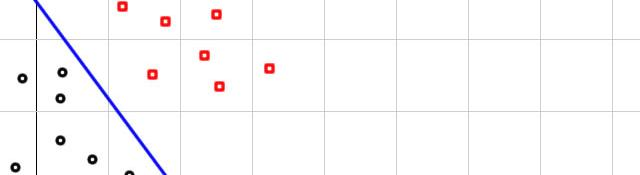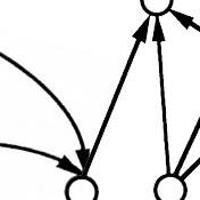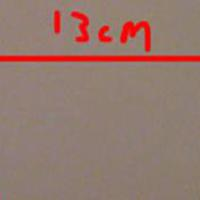## A single neuron Dictomizer

Okay, so we'll get into actually using a neural network right now! The idea is to create what is called a "dictomizer".

A dictomizer can differentiate between two "classes" of things. For example, checking if something is "human" or "not human". Or maybe checking if a book is "printed" or "hand written".

The examples I just gave are really complex. So we'll start off with something simple. The opening image at the top is what we'll try to create. We won't go into "learning". We'll just "hardwire" the neural network to differentiate between two classes.

## Working through an example

The red squares on the cartesian plane (the graph) for one "class", and the black circles form another class. Now, for each point, we have two inputs. The X coordinate, and the Y coordinate. So that fixes the number of inputs in our neural network.

Next, we just need to differentiate between two classes. So just a zero/one output is sufficient. Thus, the output is set to either a zero or a one.

Next we need to set the weights of each input. This is the key idea. This is what all future posts will be based on.Consider the above picture. The inputs x1 and x2 are the coordinates of the point. These inputs go into the "processing unit" where they are summed up based on their weights (which we need to figure out). This sum is taken through a mathematical function (which we need to figure out as well). And then it thresholded with a value T. And then we get the final output.

Now, multiple things will happen at once. This is the overall operation that we're doing:

• f(w1x1 + w2x2) < T then the output is 0
• f(w1x1 + w2x2) >= T then the output is 1

Here, f is the mathematical function. Now, if we rearrange these equations,

• f(w1x1 + w2x2) -T < 0 then the output is 0
• f(w1x1 + w2x2) - T>= 0 then the output is 1

Now, if we use f(x) = x, then these look like equations of a line (ax + by - c = 0):

• w1x1 + w2x2 - T< 0 then the output is 0
• w1x1 + w2x2 - T>= 0 then the output is 1

This is the equation of the blue line in the opening image. So now you can use geometry to figure out the equation of the blue line. And the coefficients of x and y will be weights. And the constant will be the threshold value T.

## Higher dimensions

This example was just for a 2 dimensional input (that is, there were two inputs). You can similarly have higher dimensions. 3D. 4D. 29D. You name it. It might get geometrically hard to visualize a 29D input, but yes, it can happen. It'll just have a 28D "hyperplane" (just like the 2D case had a 1D line).

These higher dimensions are used in more complex dictomizers. Like the examples I gave initially. You can give certain parameters like "distance between eyes", "length of nose", "height", "weight", "number of bones", "number of limbs", "number of teeth", and "skin surface area". These inputs can be used to check if the given object is "human" or "not human". This would be a 8D neural network.

## More examples

Next, we'll work on some more concrete examples. We'll create the OR/AND gates using just a single neuron.

#### Related posts#### 7 unique neural network architectures#### All about biological neurons#### Calibrating a camera: Theory

Utkarsh Sinha created AI Shack in 2010 and has since been working on computer vision and related fields. He is currently at Microsoft working on computer vision.